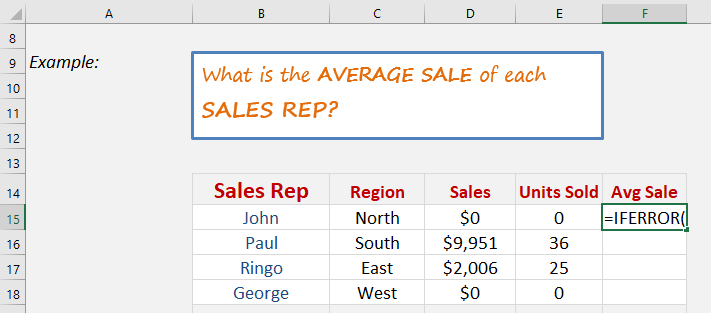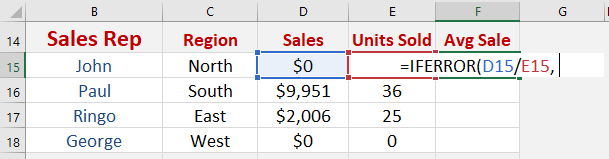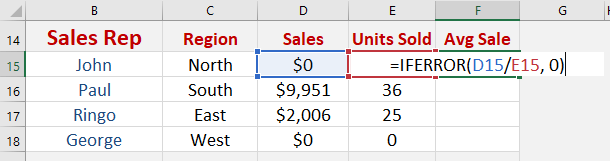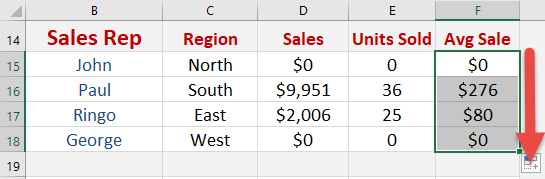What does it do?

It returns a value that you set if a formula has an error

Formula breakdown:

=IFERROR(Value,Value if Error)

What it means:

=IFERROR(The Formula,What do you want to show if The Formula has an error?)

If you have a calculation that results in an error like, #N/A, #VALUE!, #REF!, #DIV/0!, #NUM!, #NAME?, then you can clean it up by using the IFERROR function which allows you to replace the error it with a 0 or a blank cell.We want to get the average sale of each record. However we need to handle division by zero errors gracefully.

STEP 1: We need to enter the IFERROR function in a blank cell:

## =IFERROR(STEP 2: The IFERROR arguments:

## Value

What is the formula?

We need to enter the formula first to calculate the average sale.

## =IFERROR(D15/E15,## Value_if_error

What value should be displayed if there is an error in the formula?

We want “0” to be displayed if there is an error

## =IFERROR(D15/E15, 0)Apply the same formula to the rest of the cells by dragging the lower right corner downwards.You now have all of results!

How to Use the IfError Formula in Excel